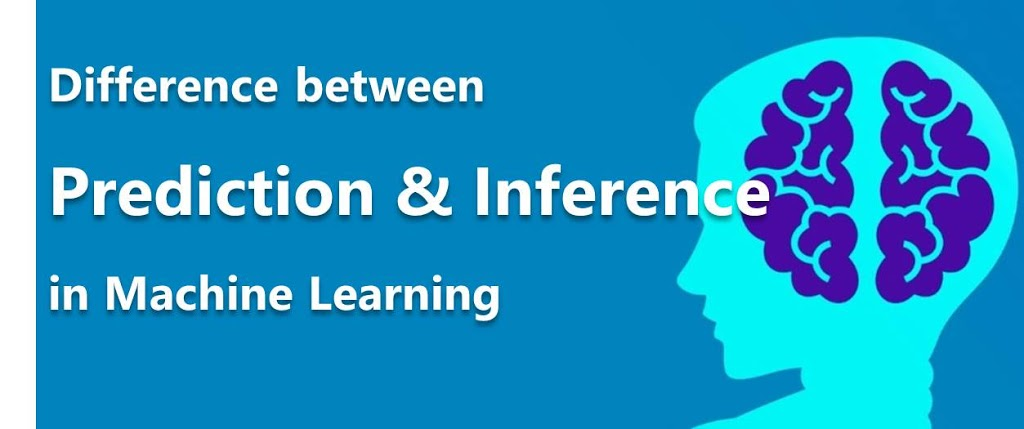April 13, 2021# Difference Between Prediction and Inference in Machine Learning

Hello friend, I’ll like to share with you this brief explanation of the difference between Prediction and Inference. They appear similar, to us researchers and data scientists, the difference should be well-drawn out.
Lets begin with Prediction

### 1. What is Prediction?

Let’s illustrate with an example.
Assuming an organization wants to conduct a marketing survey. The objective of the survey is to determine the response of people in a given area to an advertising campaign being carried out.
The company gathers the demographic features of the particular area the campaign is to be conducted. This features make up a feature set of the predictors (variables). These variables may include:

• population density
• average education level
• class of people
• area type(rural, sub-urban, urban)
• media type to be used etc

The company would like to know if the campaign would either succeed or fail, where success is measured by the response of the people to the campaign based on certain threshold.
The situation like this where the objective is to predict either success or failure (classification) given a set of input variables is an example of prediction modelling.
In this case the focus is not to understand the relationships between individual predictor variable and the output.

### 2. What is Inference?

Still using the advertising campaign example. If the objective of the survey is to determine the relationship between each individual prediction and the output this would be inference. In this case the following questions would be answered:

• Which media type have most effect on the response
• How does the population density affect the  outcome
• What class of people respond most to the campaign

In this case, the goes is not just to get a classification of success or failure but to infer relationships between predictors.
Let’s look at the  mathematical model

### 3. The Mathematical Model

Let the input predictor variables be X1, … Xp. and the target variable Y.  The we would be interested in estimating how the output Y is affected by variation in X.
The goal is the create a relationship between X and Y such that
f(X) = Y
First we assume a simple approach and the would be that the model is linear. Then we use a parametric method.
Assuming the function f(X) is linear, then we have:

Next we then train the model by using a procedure that finds the values of the βs such that:

Here you can see that the equivalent sign is used which means that the predicted that there exist some error value incurred in the process which we would like to minimize.
More details on this is discussed in Linear Models of  Regression#### kindsonthegenius

View all posts by kindsonthegenius →
0 0 vote
Article Rating
Subscribe
Notify of0 Comments
Inline Feedbacks
View all comments